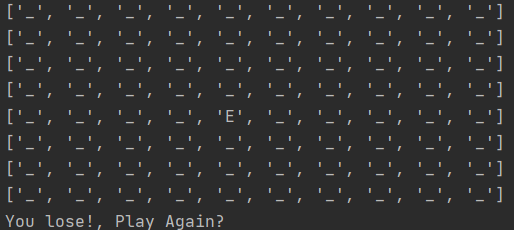# Agents! how do they work?# Program Design

## Classes:

Board

Board is the most sophisticated class within the program , its functionality is actually split in two separate data structures:

1. To display the board , a nested list (10×10) that displays the player an enemy as string elements (“P”,“E”)
`def __init__(self, player=None, enemy=None):self.main_board =[["_", "_", "_", "_", "_", "_", "_", "_", "_","_"],                  ["_", "_", "_", "_", "_", "_", "_", "_", "_","_"],                  ["_", "_", "_", "_", "_", "_", "_", "_", "_","_"],                  ["_", "_", "_", "_", "_", "_", "_", "_", "_","_"],                  ["_", "_", "_", "_", "_", "_", "_", "_", "_","_"],                  ["_", "_", "_", "_", "_", "_", "_", "_", "_","_"],                  ["_", "_", "_", "_", "_", "_", "_", "_", "_","_"],                  ["_", "_", "_", "_", "_", "_", "_", "_", "_","_"],                  ["_", "_", "_", "_", "_", "_", "_", "_", "_","_"],                  ["_", "_", "_", "_", "_", "_", "_", "_", "_","_"]]`

2. A graph data structure in the background, that stores the board as a collection of interconnected node objects. The graph stores the positions of each node and also the neighbor positions to each node in the graph, this information is the base for A* implementation

`"""Node Class stores node information for each node in the board"""class Node():    def __init__(self, coordinates):        self.coordinates = coordinates        self.neighbors = []"""Create neighbors creates and stores the neigbhor information for all the elements in the board """    def create_neighbors(self, board_nodes):        directions = [(1, 0), [0, 1], [-1, 0], [0, -1]]        for direction in directions:            neighbor_coordinates = (self.coordinates+direction, self.coordinates+direction)            for j in board_nodes:                if j.coordinates == neighbor_coordinates:                    self.neighbors.append(j)`

Board keeps references to both the Player and Enemy objects as well as a list with all the nodes created for each board position. Note that the node stores te coordinates of the node within the board, so we just need a single reference to obtain and update the position of an agent within the environment

`self.nodes = []self.player = playerself.enemy = enemy`

Finally , board positions the initial Player and Enemy (Player always starts for a fixed position, Enemy from a random one), creates all the nodes in the board and their neighbor connectivity and displays the game state

`def place_player(self):    player_row_index = 9    player_column_index = 5    for i in self.nodes:        if i.coordinates == (player_row_index, player_column_index):            player_node = i    player = Agent.Player(player_row_index, player_column_index, player_node)    player.node = player_node    self.player = player    self.main_board[player.row][player.column] = player.tagdef place_enemy(self):    enemy_row_index = random.randint(0, 9)    enemy_column_index = random.randint(0, 9)    for i in self.nodes:        if i.coordinates == (enemy_row_index, enemy_column_index):            enemy_node = i    enemy = Agent.Enemy(enemy_row_index, enemy_column_index, enemy_node)    self.main_board[enemy.row][enemy.column] = enemy.tag    self.enemy = enemydef create_nodes(self):    board_height = len(self.main_board)    board_width = len(self.main_board)    for x in range(board_width):        for y in range(board_height):            node = Node((x, y))            self.nodes.append(node)def create_node_neighbors(self):    for node in self.nodes:        node.create_neighbors(self.nodes)def display_board(self):    for i in self.main_board:        print(i)`

Agent

The agent class is rather simple , it only needs a tag , to designate it as Player (“P”) or Enemy (“E”). It also needs a reference to the graph’s node, and that’s it!

`class Agent():    def __init__(self, row_index=None, column_index=None, tag="", node=None):        self.position = [row_index, column_index]        self.node = node        self.tag = tag`

## The Game Loop

The basic operations of the game are summarized with this loop

Of these, the method that results of most interest is the Move Enemy method. This is where the A* algorithm and the graph implementation come into play.

I don’t want to get into a full explanation of how A* is implemented, a magnificent explanation can be found here :

My implementation is almost identical to the basic form of A* given by Red Blob Games , a summarized explanation on how the algorithm works is:

1. From the enemy starting position , the entirety of the graph is traversed , recording the position of every node relative to that of the enemy, in such a way that all the nodes in the graph have a recorded path to the enemy’s position
2. Knowing the player’s position, a path is constructed from the node where the player is known to be , connecting it to the enemy position. A list of nodes is generated forming the path that connects the two positions.
3. The enemy then moves to one of the nodes in the constructed path , updating his position to be closer to the player.

The whole process is a relatively short program, notice the implementation of a Queue , to enqueue and dequeue the neighbor nodes as we traverse the graphs

`def move_enemy(board):    player_node = board.player.node    enemy_node = board.enemy.node    """    Enemy agent scans the board     """    frontier = Queue.Queue()    frontier.enqueue(enemy_node)    came_from={}    came_from[enemy_node] = None    while not frontier.is_empty():        current = frontier.dequeue()        for nxt in current.neighbors:            if nxt not in came_from:                frontier.enqueue(nxt)                came_from[nxt] = current    """    Enemy Agent builds the path towards the player     """    current = player_node    path = []    while current != enemy_node:        path.append(current)        current = came_from[current]    path.append(enemy_node)    path.reverse()    for i in path:        print(i.coordinates)    """    Updates the enemy position in the board based on the constructed path towards the player    """    board.main_board[board.enemy.node.coordinates][board.enemy.node.coordinates] = "_"    board.enemy.node = path    board.main_board[board.enemy.node.coordinates][board.enemy.node.coordinates] = "E"    board.display_board()    return board`